Author: Oscar Cronquist Article last updated on November 01, 2021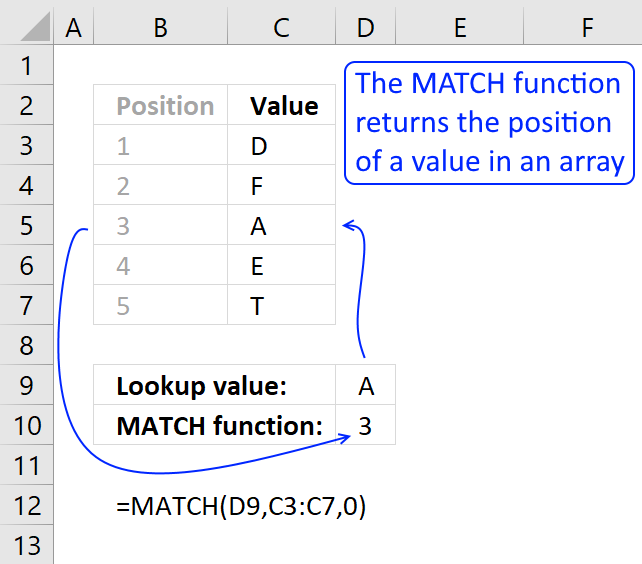The MATCH function returns the relative position of an item in an array or cell reference that matches a specified value in a specific order.

The formula in cell D10 searches for value "A" in cell range C3:C7 and finds an exact match in cell C5. The relative position of C5 in cell range C3:C7 is 3, shown in column B.

The MATCH function is probably one of the most used Excel functions, it is more versatile combined with the INDEX function than the VLOOKUP function.

## 1. MATCH Function Syntax

MATCH(lookup_value, lookup_array, [match_type])

## 2. MATCH function arguments

 lookup_value Required. Is the value you use to find the value you want in the array, a number, text or logical value, or a reference to one of these. lookup_array Required. Is a contiguous range of cells containing possible lookup values, an array of values, or a reference to an array. [match_type] Optional. How to match, a number -1,0,1. If omitted default value is number 1.

match_type

 -1 Finds the largest value that is less than or equal to lookup_value. Lookup_array must be in ascending order. 0 Finds the value that is exactly equal to lookup_value. Lookup_array can be in any order 1 Finds the smallest value that is greater than or equal to lookup_value. Lookup_array must be sorted in a descending order

Be careful with the third argument [match_type], remember to use 0 (zero) in the third argument if you want to find an exact match which you almost always want to.

If you don't use 0 (zero) in the third argument, the values in the lookup_array argument must be sorted in an ascending or descending order based on the lookup_array number you choose.

• The lookup_array must be  a one dimensional horizontal or vertical cell range
• The lookup_value can also be an array, one or two dimensional. (array formula)
• The lookup_array can be one value. (array formula)
• You can use other Excel functions in the lookup_value argument.
• You can use other Excel functions in the lookup_array argument as long as they return a one-dimensional array.
• I use match_type = 0 almost all the time.

Below are examples demonstrating what the formula returns using different arguments.

## 3. Lookup_array in ascending orderThis example shows what happens and what is required if you use 1 as the [match_type] argument. Cell range C19:C21 har numbers sorted in an ascending order, which is required to get reliable results.

In this setup, the MATCH function finds the largest value that is less or equal to the lookup_value. You can use text values as well, however, make sure they are sorted in ascending order.

=MATCH(C17, C19:C21, 1)

becomes

MATCH(2, {1; 5; 10; 12}, 1)

{1; 5; 10; 12} is an array of values separated by semicolons which means they are located in a row each.

[Match_type] 1 - Find the largest value {1; 5; 10; 12} that is less or equal to lookup_value which is 2.

MATCH(2, {1; 5; 10; 12},1)

There is only one value that is less or equal to the lookup value and that number is 1. The relative position of number 1 in array {1; 5; 10; 12} is 1. 1 is returned in cell D14.

Note, make sure your values are sorted in ascending order in this setup.

## 4. Example 2 - Lookup_array in any order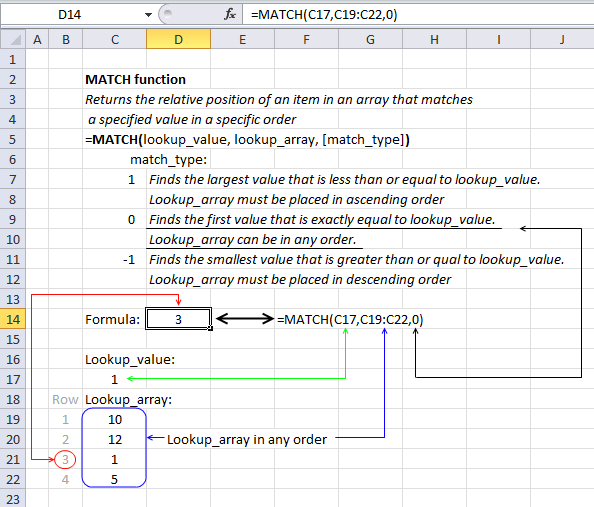This setup is what I recommend using and is what I am using myself the most. [match_type] is 0 (zero) which allows you to have the lookup_array in any order you want.

The image above demonstrates the following formula in cell D14:

=MATCH(C17, C19:C21,0)

The first argument (lookup_value) is in cell C17, and the second argument  (lookup_array) is in cell range C19:C22.

=MATCH(C17, C19:C21,0)

becomes

MATCH(1, {10; 12; 1; 5},0)

{10; 12; 1; 5} is an array of values separated by semicolons which means they are located in a row each.

MATCH(1, {10; 12; 1; 5},0)

Match_type 1 - Find the first value {10; 12; 1; 5} that is exactly equal to lookup_value (1)

MATCH(1, {10; 12; 1; 5},0)

The relative position of number 1 in array {10; 12; 1; 5} is 3. 3 is returned in cell D14.

## 5. Lookup_array in descending order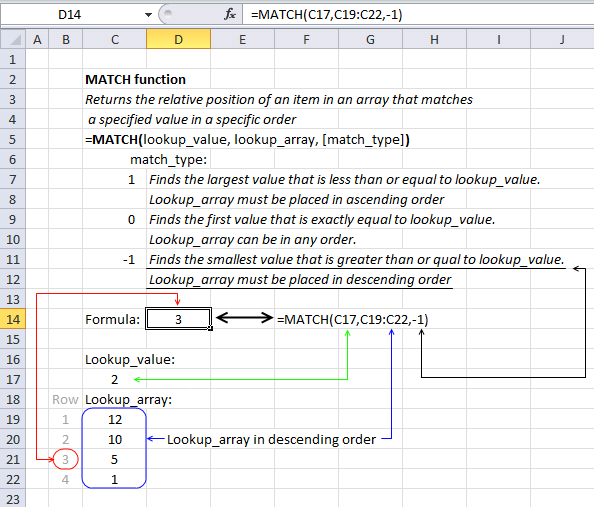The following formula has -1 as the third argument [match_type], the lookup_array must be sorted in descending order for this setup to work.

In this case, the MATCH function finds a value in cell range C19:C22 that is larger than or equal to the lookup_value which is specified in cell C17.

=MATCH(C17, C19:C21,-1)

becomes

MATCH(2, {12; 10; 5; 1},-1)

Match_type 1 - Find the smallest value {12; 10; 5; 1} that is greater than or equal to lookup_value (2)

MATCH(2, {12; 10; 5; 1},-1)

The relative position of number 5 in the following array {12; 10; 5; 1} is 3. 3 is returned in cell D14.

## 6. How to use the MATCH function in an array formula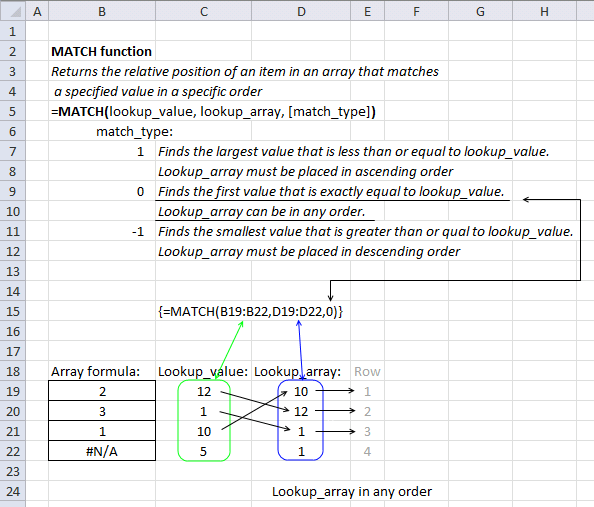This example demonstrates what happens if you use multiple values in the first argument lookup_value. The MATCH function returns an array of values and you are required to enter the formula as an array formula.

1. Select cell range B19:B22.
2. Copy the formula below and paste to cell or formula bar.
3. Press and hold CTRL + SHIFT simultaneously.
4. Press Enter once.

Excel adds curly brackets to the formula automatically, don't enter these characters yourself.

=MATCH(C19:C22, D19:D22,0)

becomes

MATCH({12;1;10;5}, {10; 12; 1; 1},0)

Match_type 0 (zero) finds the first value {10; 12; 1; 1} that is exactly equal to lookup_value {12;1;10;5}

Lookup value 12 is the second value in the lookup_array {10; 12; 1; 1}.

Lookup value 1 is the third value in the lookup_array {10; 12; 1; 1}.

Lookup value 10 is the first value in the lookup_array {10; 12; 1; 1}.

Lookup value 5 is not found in the lookup_array {10; 12; 1; 1}.

{2; 3; 1; #N/A} is returned in cell range B19:B22.

Update!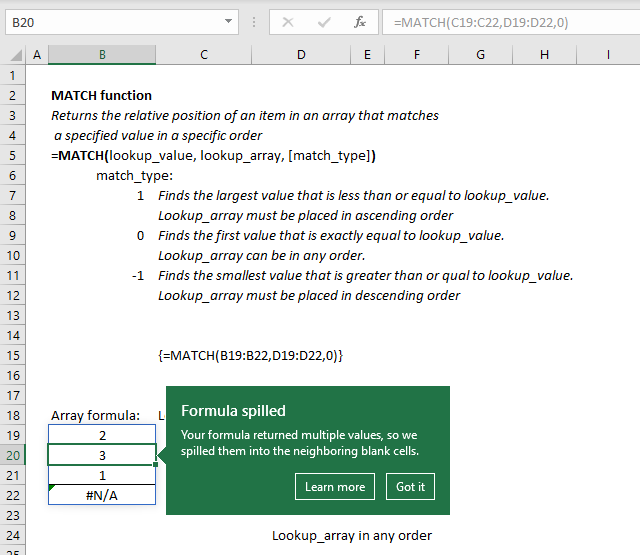Dynamic arrays were recently introduced to Excel 365 subscribers, they are different from regular array formulas. You don't need to enter the formula as an array formula, simply enter them as a regular formula.

Excel knows that the formula returns multiple values and extends the selection automatically, this is called spilled array behavior.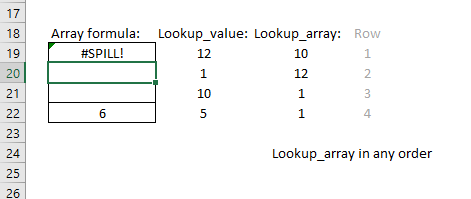Excel returns a #SPILL! error in case there are values in cells below that prevent all array values to be displayed.

## 7. How to do a partial match - Wildcard characters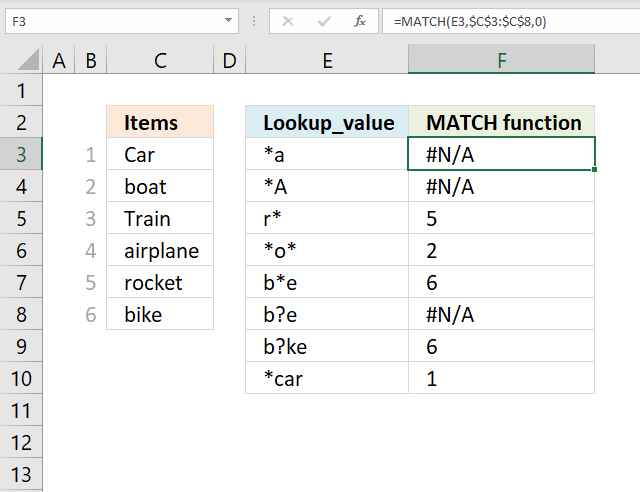The asterisk character allows you to perform wilcard searches, it represents 0 (zero) to any number of characters. If this is unclear then check out the examples below.

### 7.1 Match cell that ends with condition

The formula in cell F3 looks for values in cell range C3:C8 that match any character and then ends with an a. No cell value in cell range C3:C8 matches that condition so the function returns #N/A error.

=MATCH(E3,\$C\$3:\$C\$8,0)

The formula in cell F4 is similar to cell F3 but it ends with a capital letter A. No values match that condition either and the formula returns a #N/A! error which means that the value is not available.

Formula in cell F10:

=MATCH(E10,\$C\$3:\$C\$8,0)

Cell E10 contains "*car" which matches the first value in cell range C3:C8, remember that the asterisk also matches no character. The formula returns 1.

### 7.2 Match cell that begins with conditionThe next formula looks for a value that starts with an r and can contain any number of characters after that. Cell E5 contains r*.

Formula in cell F5:

=MATCH(E5,\$C\$3:\$C\$8,0)

The formula returns 5 which is the relative position of value "rocket" in cell range C3:C8, note that the condition would also have matched value  "Rocket" and "r" and "R". The asterisk means no character up to any number of any character.

### 7.3 Match cell that contains stringFormula in cell F6:

=MATCH(E6,\$C\$3:\$C\$8,0)

The formula in cell F6 looks for a value that matches *o* which means any number of characters before and after o. The first value that matches that condition is found in position 2 which is "boat", however, there are more values that match. Value "rocket" would have matched the condition but the MATCH function returns only the number of the first value found in cell range C3:C8.

### 7.4 Match cell that begins with string and ends with another string - any number of characters in betweenFormula in cell F7:

=MATCH(E7,\$C\$3:\$C\$8,0)

Cell E7 contains b*e which means that the value must begin with a b or B and must end with a e or E. Only one value matches that condition which is "bike". 7 is returned in cell F7.

### 7.5 Match cell that begins with string and ends with another string - a single character in betweenThe question mark character is different than the asterisk character, it matches only a single character.

Formula in cell F8:

=MATCH(E8,\$C\$3:\$C\$8,0)

Cell E8 contains b?e and the MATCH function cant find a value in cell range C3:C8 that starts with a "b", matches any single character, and ends with an "e".

Value "bike" has two characters between b and e. The formula returns #N/A! in cell F8.

Formula in cell F9:

=MATCH(E9,\$C\$3:\$C\$8,0)

Cell E9 contains "b?ke" and matches cell C6 which is the last value in cell range C3:C8. The formula returns 6.

## 8. VBA Example

The following macro uses the MATCH function to find the position of the lookup value in cell range C5:C8. It then displays a message box containing that position.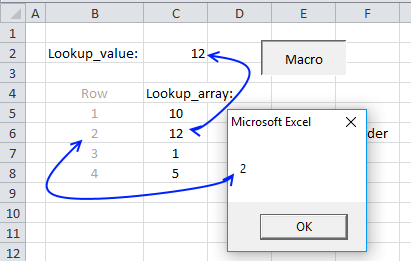### 8.1 VBA code

```Sub VBA_MATCH()
MsgBox Application.WorksheetFunction.Match(Range("C2"), Range("C5:C8"), 0)
End Sub
```

## 9. How to do a case sensitive match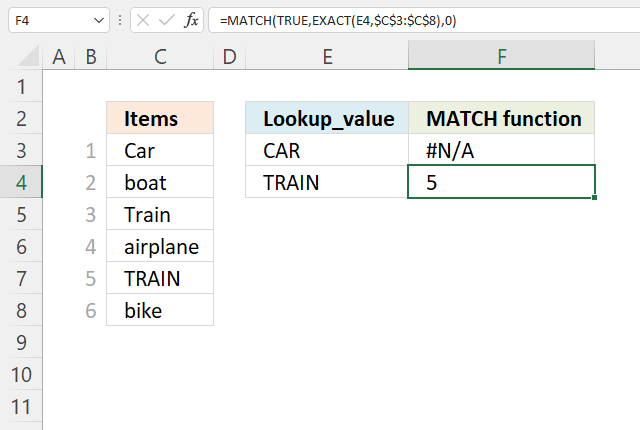The formulas in column F perform a case-sensitive match, the lookup values are in column E and the lookup array is in cell range C3:C8.

Array formula in cell F4:

=MATCH(TRUE, EXACT(E4, \$C\$3:\$C\$8), 0)

The result is a number representing the position of the lookup value in the lookup array.

### 9.1 Explaining formula in cell F4

#### Step 1 - Case sensitive comparison

The EXACT function performs a case-sensitive comparison. The result is a boolean value TRUE or FALSE.

EXACT(E4,\$C\$3:\$C\$8)

becomes

EXACT("TRAIN",{"Car";"boat";"Train";"airplane";"TRAIN";"bike"})

and returns

{FALSE; FALSE; FALSE; FALSE; TRUE; FALSE}.

#### Step 2 - Match boolean value TRUE

MATCH(TRUE, EXACT(E4, \$C\$3:\$C\$8), 0)

becomes

MATCH(TRUE, {FALSE; FALSE; FALSE; FALSE; TRUE; FALSE}, 0)

and returns 5 in cell F4.

## 11. Get Excel file

### Get the Excel fileMatch-function.xlsx